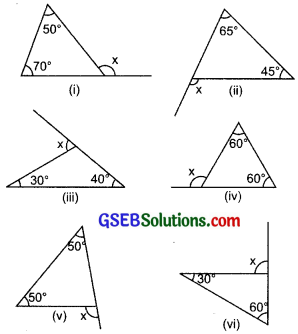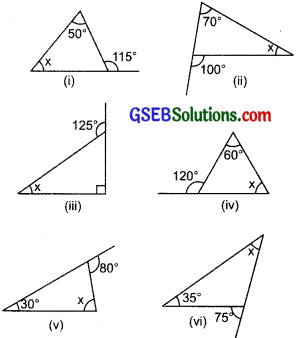# GSEB Solutions Class 7 Maths Chapter 6 The Triangles and Its Properties Ex 6.2

Gujarat Board GSEB Textbook Solutions Class 7 Maths Chapter 6 The Triangles and Its Properties Ex 6.2 Textbook Questions and Answers.

## Gujarat Board Textbook Solutions Class 7 Maths Chapter 6 The Triangles and Its Properties Ex 6.2Question 1.
Find the value of the unknown exterior angle x in the following diagrams:Solution:
(i) ∵ Interior opposite angles are 50° and 70°.
∴ Using the exterior angle property of a triangle, we have
[Exterior angle] = [Sum of the interior opposite angles]
or x = 50° + 70° = 120°.

(ii) ∵ Interior opposite angles are 65° and 45°.
∴ Using the relation,
[Exterior angle] = [Sum of the interior opposite angles]
We have x = 65° + 45°
or x = 110°.

(iii) ∵ Interior opposite angles are 30° and 40°.
∴ Using the relation,
[Exterior angle] = [Sum of the interior opposite angles]
We have x = 30° + 40°
or x = 70°.

(iv) ∵ The interior opposite angles are 60° and 60°.
∴ Using the relation,
[Exterior angle] = [Sum of interior opposite angles]
We have x = 60° + 60°
or x = 120°.

(v) ∵ The interior opposite angles are 50° and 50°.
∴ Using the relation,
[Exterior angle] = [Sum of the interior opposite angles]
We have x = 50° + 50°
or x = 100°.

(vi) ∵ The interior opposite angles are 30° and 60°.
We have x = 30° + 60°
or x = 90°.Question 2.
Find the value of the unknown interior angle x in the following figures:Solution:
(i) Exterior angle =115°
One of the interior opposite angles = 50°
Using the relation,
[Sum of the interior opposite angles] = [Exterior angle]
We have x + 50° = 115°
or x = 115° – 50°
or x = 65°.
Thus, the measure of the unknown interior angle is 65°.

(ii) ∵ Exterior angle = 100°
Interior opposite angles are 70° and x.
∴ Using the relation,
[Sum of the interior opposite angles] = [Exterior angle]
We have 70° + x = 100°
or x = 100° – 70°
or x = 30°
∴ The required measure of the unknown interior opposite angle is 30°.

(iii) ∵ Exterior angle = 125°
Interior opposite angles are 90° and x.
∴ Using the relation,
[Sum of the interior opposite angles] = [Exterior angle]
We have x + 90° = 125°
or x = 125° – 90° = 35°
Thus, the value of unknown interior opposite angle is 35°.

(iv) ∵ Exterior angle = 120°
Interior opposite angles are 60° and x.
∴ Using the relation,
[Sum of the interior opposite angles] = [Exterior angle]
We have x + 60° = 120°
or x = 120° – 60° = 60°
Thus, the measure of the unknown interior opposite angle is 60°.

(v) ∵ Exterior angle = 80°
Interior opposite angles are 30° and x.
∴ Using the relation,
[Sum of the interior opposite angles] = [Exterior angle]
We have: 30° + x = 80°
or x = 80° – 30° = 50°
Thus, the measure of the unknown interior angle is 50°.

(vi) ∵ Exterior angle = 75°
Interior opposite angles are x and 35°.
∴ Using the relation,
[Sum of the interior opposite angles] = [Exterior angle]
We have x + 35° = 75°
or x = 75° – 35°
or x = 40°
Thus, the measure of the interior opposite angle is 40°.